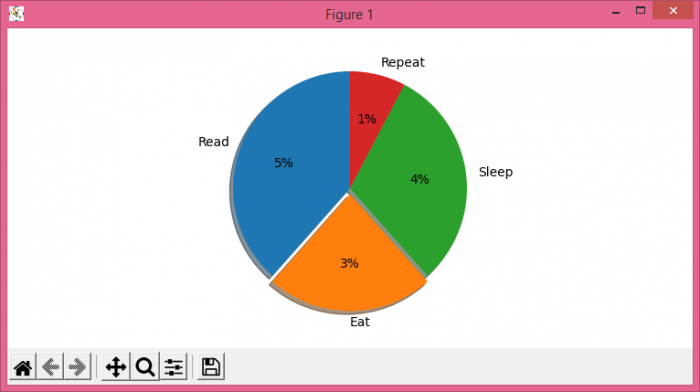# How to have actual values in Matplotlib Pie Chart displayed?

To have actual or any custom values in Matplotlib pie chart displayed, we can take the following steps −

• Set the figure size and adjust the padding between and around the subplots.
• Make lists of labels, fractions, explode position and get the sum of fractions to calculate the percentage
• Make a pie chart using labels, fracs and explode with autopct=lambda p: <calculation for percentage>.
• To display the figure, use show() method.

## Example

import matplotlib.pyplot as plt

plt.rcParams["figure.figsize"] = [7.50, 3.50]
plt.rcParams["figure.autolayout"] = True

labels = ('Read', 'Eat', 'Sleep', 'Repeat')

fracs = [5, 3, 4, 1]
total = sum(fracs)
explode = (0, 0.05, 0, 0)

plt.pie(fracs, explode=explode, labels=labels,
autopct=lambda p: '{:.0f}%'.format(p * total / 100),
plt.show()